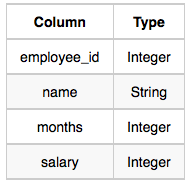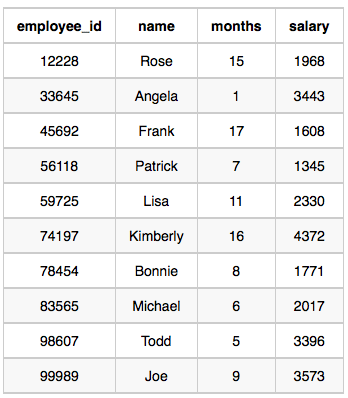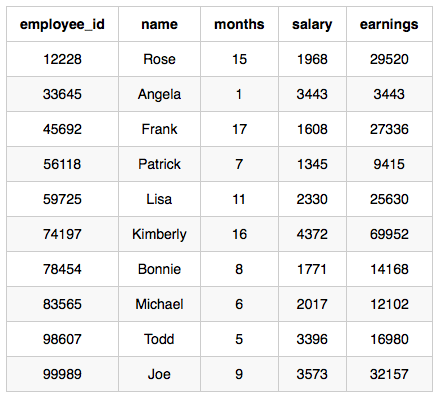# Top Earners Hackerrank MySQL and aggregation

##### Top Earners Hackerrank MySQL and aggregation

We define an employee’s total earnings to be their monthly salary X months worked, and the maximum total earnings to be the maximum total earnings for any employee in the Employee table. Write a query to find the maximum total earnings for all employees as well as the total number of employees who have maximum total earnings. Then print these values as 2 space-separated integers.

Input Format

The Employee table containing employee data for a company is described as follows:where employee_id is an employee’s ID number, name is their name, months is the total number of months they’ve been working for the company, and salary is their monthly salary.

Sample InputSample Output

69952 1
Explanation

The table and earnings data is depicted in the following diagram:The maximum earnings value is 69952. The only employee with earnings 69952 is Kimberly, so we print the maximum earnings value (69952) and a count of the number of employees who have earned \$69952 (which is 1) as two space-separated values.

##### Solution

Mysql

``select (salary * months)as earnings ,count(*) from employee group by 1 order by earnings desc limit 1``

Oracle

``````select * from (
select months * salary, count(*)
from employee
group by months * salary
order by 1 desc
) where rownum = 1;``````

MS SQL

``select (select cast(max(salary*months) as varchar) from employee)+' '+(select cast(count(*) as varchar) from employee where salary*months =(select max(salary*months) from employee))``
``select salary*months,count(1) as empcount from employee group by salary*months order by salary*months desc offset 0 rows fetch next 1 rows only``

Weather Observation Station 2 Hackerrank

Query the following two values from the STATION table:

The sum of all values in LAT_N rounded to a scale of 2 decimal places.
The sum of all values in LONG_W rounded to a scale of 2 decimal places.
Input Format

The STATION table is described as follows:where LAT_N is the northern latitude and LONG_W is the western longitude.

Output Format

Your results must be in the form:

lat lon
where lat is the sum of all values in LAT_N and lon is the sum of all values in LONG_W. Both results must be rounded to a scale of 2 decimal places.

``select round(sum(lat_n),2) , round(sum(long_w), 2) from station``

### hasectic

A web developer(Front end and Back end), and DBA at csdamu.com. Currently working as Salesforce Developer @ Tech Matrix IT Consulting Private Limited. Check me @about.me/s.saifi